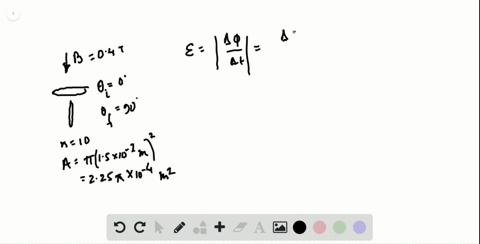🎉 The Study-to-Win Winning Ticket number has been announced! Go to your Tickets dashboard to see if you won! 🎉View Winning Ticket### The surface of the coil of wire discussed in Prob…

06:26Johns Hopkins University
Problem 36

# Generator for space station Astronauts on a space station decide to use Earth's magnetic field to generate electric current. Earth's $\vec{B}$ field in this region has the magnitude of $3.0 \times 10^{-7} \mathrm{T}$ . They have a coil that rotates $90^{\circ}$ in 1.2 $\mathrm{s}$ . The area inside the coil measures 5000 $\mathrm{m}^{2}$ . Estimate the number of loops needed in the coil so that during that $90^{\circ}$ turn it produces an average induced emf of about 120 V. Indicate any assumptions youmade. Is this a feasible way to produce electric energy?

## Discussion

You must be signed in to discuss.

## Video Transcript

in this question, we have been given a very interesting scenario where astronauts and space are trying to use induction to produce electricity. So in this case we are given the Earth's magnetic field in that region to be three times 10 to the negative seventh test. Sula and the astronauts are equipped with a coil that rotates 90 degrees in 1.2 seconds. So that tells me the change in angle is 90 degrees overall. And how much time did that take That took 1.2 seconds. They also tell us the area inside the coil measures 5000 meters squared, so area is 5000 meters squared. Now, that is a pretty large oil. Um, and here we're going to estimate the number of loops needed in the coils so that during the 90 degree turn, it produces an average induced E m f about 120 volts. So they do tell us about did you tell us the target? Yemma And they are asking for us for the number of turns. Um, so this we are here. We are going to use Faraday's law of induction again, and that is equal to end times to change in magnetic flux over time. So in this case, we know that, um, Ennis, what we're looking for and we do have be we do have a what is changing is the angle. So remember, if I is equal to be a co sign of the angle So for the purpose of simplicity, I am just going to, um, say that the final angle of zero and the initial angle is 90 degrees. Either way, the changes 90 degrees, and that's what they're asking for right all over the changing time. And to simplify this further. I know co sign of zero is one, and the coastline of 90 is zero, So that will make our calculation much simpler. We have The MF is equal to n B A divided by Delta t So now we just have to solve for n and so and we'll equal to a e m f times change in time over B times A. So let's go ahead and plug in everything. We have 1 20 bolts times the changing time, which is 1.8 seconds divided by B, which is three times 10 to the negative. Seventh, uh, times a, which is five 1000 and plugging all of that into the calculator. We have how many turns 96,000 turns, and that's how you saw for the unknown in this question.